# A Review of 2022

Author

Steven P Sanderson II, MPH

Published

January 3, 2023

2022 A Year in Review

The year 2022 was a big year for me. I did a lot of coding, a lot more than I typically do. The biggest push came personally in my ongoing development of my R packages that are in the healthyverse. To use the healthyverse simply do so in the familiar fashion:

``````install.packages("healthyverse")
library(healthyverse)``````

Here are links to all of the packages:

In order to start looking at some of the data that pertains to 2022 lets first get the data from the CRAN logs. Since I do this daily already, I can simply use the rds file I already have. I am going to go through the motions though, in case others might want to do something similar. The functions I am using to get the data can be found here

Now lets get that data!

``````library(tidyverse)
library(lubridate)
source("01_scripts/get_data_functions.R")
source("01_scripts/data_manipulation_functions.R")
source("01_scripts/mapping_functions.R")

get_cran_data()
get_package_release_data()
csv_to_rds()``````

Ok now that we have our data, lets ensure that we are only using the year 2022. We can do this by filtering out data by time with the timetk package.

Now lets filter our data below, some pre-processing may need to take place.

``````library(timetk)
filter_by_time(
.date_var = date,
.start_date = "2022",
.end_date = "2022"
)

glimpse(data_tbl)``````
``````Rows: 27,779
Columns: 11
\$ date      <date> 2022-01-01, 2022-01-01, 2022-01-01, 2022-01-01, 2022-01-01,…
\$ time      <Period> 7H 9M 18S, 1H 40M 38S, 1H 40M 46S, 1H 41M 4S, 10H 52M 47S…
\$ date_time <dttm> 2022-01-01 07:09:18, 2022-01-01 01:40:38, 2022-01-01 01:40:…
\$ size      <int> 4866327, 1032739, 989862, 1028890, 13101, 14533, 14548, 1310…
\$ r_version <chr> NA, NA, NA, NA, NA, NA, NA, NA, NA, NA, "4.1.2", "4.1.2", "4…
\$ r_arch    <chr> NA, NA, NA, NA, NA, NA, NA, NA, NA, NA, "i386", "i386", "i38…
\$ r_os      <chr> NA, NA, NA, NA, NA, NA, NA, NA, NA, NA, "mingw32", "mingw32"…
\$ package   <chr> "healthyR.data", "healthyR", "healthyR", "healthyR", "health…
\$ version   <chr> "1.0.1", "0.1.6", "0.1.6", "0.1.6", "1.0.1", "0.1.6", "0.1.6…
\$ country   <chr> "AU", "US", "US", "US", "US", "US", "US", "US", "US", "AU", …
\$ ip_id     <int> 52, 48, 48, 48, 6414, 3451, 487, 817, 1251, 52, 1149, 1149, …``````

Now that we have our data, we have it for the year 2022 only with a start date of 2022-01-01 and an end date of 2022-12-30.

# Package Information

The first thing we will do is look at how many downloads there were for each pacakge and it’s version.

``````library(knitr)

data_tbl %>%
count(package, version) %>%
pivot_wider(
id_cols       = version
, names_from  = package
, values_from = n
, values_fill = 0
) %>%
arrange(version) %>%
kable()``````
version healthyR healthyR.ai healthyR.data healthyR.ts healthyverse TidyDensity
0.0.1 0 88 0 0 0 993
0.0.10 0 381 0 0 0 0
0.0.2 0 91 0 0 0 0
0.0.3 0 91 0 0 0 0
0.0.4 0 141 0 0 0 0
0.0.5 0 985 0 0 0 0
0.0.6 0 1082 0 0 0 0
0.0.7 0 706 0 0 0 0
0.0.8 0 836 0 0 0 0
0.0.9 0 607 0 0 0 0
0.1.0 88 0 0 87 0 0
0.1.1 89 0 0 99 0 0
0.1.2 101 0 0 87 0 0
0.1.3 89 0 0 87 0 0
0.1.4 90 0 0 87 0 0
0.1.5 90 0 0 90 0 0
0.1.6 137 0 0 90 0 0
0.1.7 970 0 0 881 0 0
0.1.8 1080 0 0 1056 0 0
0.1.9 876 0 0 485 0 0
0.2.0 1340 0 0 487 0 0
0.2.1 0 0 0 301 0 0
0.2.2 0 0 0 541 0 0
0.2.3 0 0 0 532 0 0
0.2.4 0 0 0 153 0 0
0.2.5 0 0 0 400 0 0
1.0.0 0 0 90 0 125 408
1.0.1 0 0 3616 0 217 908
1.0.2 0 0 0 0 3028 0
1.1.0 0 0 0 0 0 445
1.2.0 0 0 0 0 0 529
1.2.1 0 0 0 0 0 347
1.2.2 0 0 0 0 0 570
1.2.3 0 0 0 0 0 593
1.2.4 0 0 0 0 0 489

Now lets see how many total downloads for the year there were for each package.

``````data_tbl %>%
count(package) %>%
kable()``````
healthyR 4950
healthyR.ai 5008
healthyR.data 3706
healthyR.ts 5463
healthyverse 3370
TidyDensity 5282
``````data_tbl %>%
select(package, version) %>%
group_by(package) %>%
distinct() %>%
mutate(release_count = n()) %>%
ungroup() %>%
select(package, release_count) %>%
distinct() %>%
set_names("Package", "Number of Releases") %>%
kable()``````
Package Number of Releases
healthyR.data 2
healthyR 11
healthyverse 3
healthyR.ai 10
healthyR.ts 16
TidyDensity 9
``````total_number_of_releases <- data_tbl %>%
select(package, version) %>%
group_by(package) %>%
distinct() %>%
mutate(release_count = n()) %>%
ungroup() %>%
select(package, release_count) %>%
distinct() %>%
summarise(total = sum(release_count, na.rm = TRUE))``````

So all in all there was a total of 27,779 downloads of all the `healthyverse` packages in 2021. There were in total 51 package releases as well.

# Graphs

Now lets graph the data out!

``````data_tbl %>%
count(package, version) %>%
ggplot(aes(x = version, y = n, alpha = 0.382)) +
geom_col(aes(group = package, fill = package)) +
facet_wrap(package ~., ncol = 2, scales = "free") +
scale_y_continuous(labels = scales::label_number(big.mark = ",")) +
theme_minimal() +
theme(legend.position = "bottom") +
labs(
subtitle = "Faceted by Package",
x = "Version",
fill = "Package"
)``````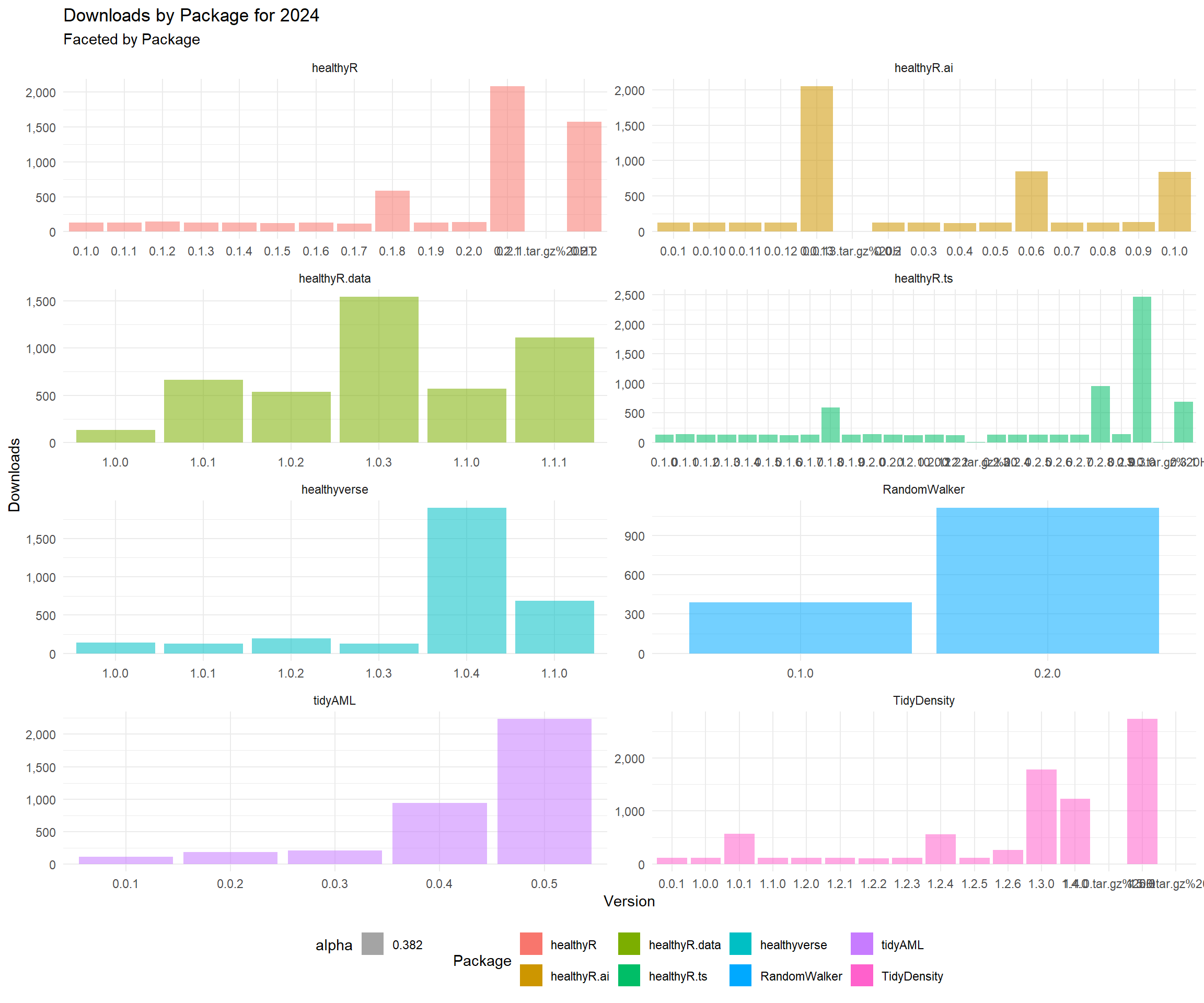``````data_tbl %>%
count(package, version) %>%
group_by(package) %>%
mutate(record = row_number()) %>%
ungroup() %>%
geom_col(aes(group = package, fill = package)) +
facet_wrap(package ~., ncol = 2, scales = "free") +
scale_y_continuous(labels = scales::label_number(big.mark = ",")) +
theme_minimal() +
theme(legend.position = "bottom") +
labs(
subtitle = "Faceted by Package",
x = "Relase Number",
fill = "Package"
)``````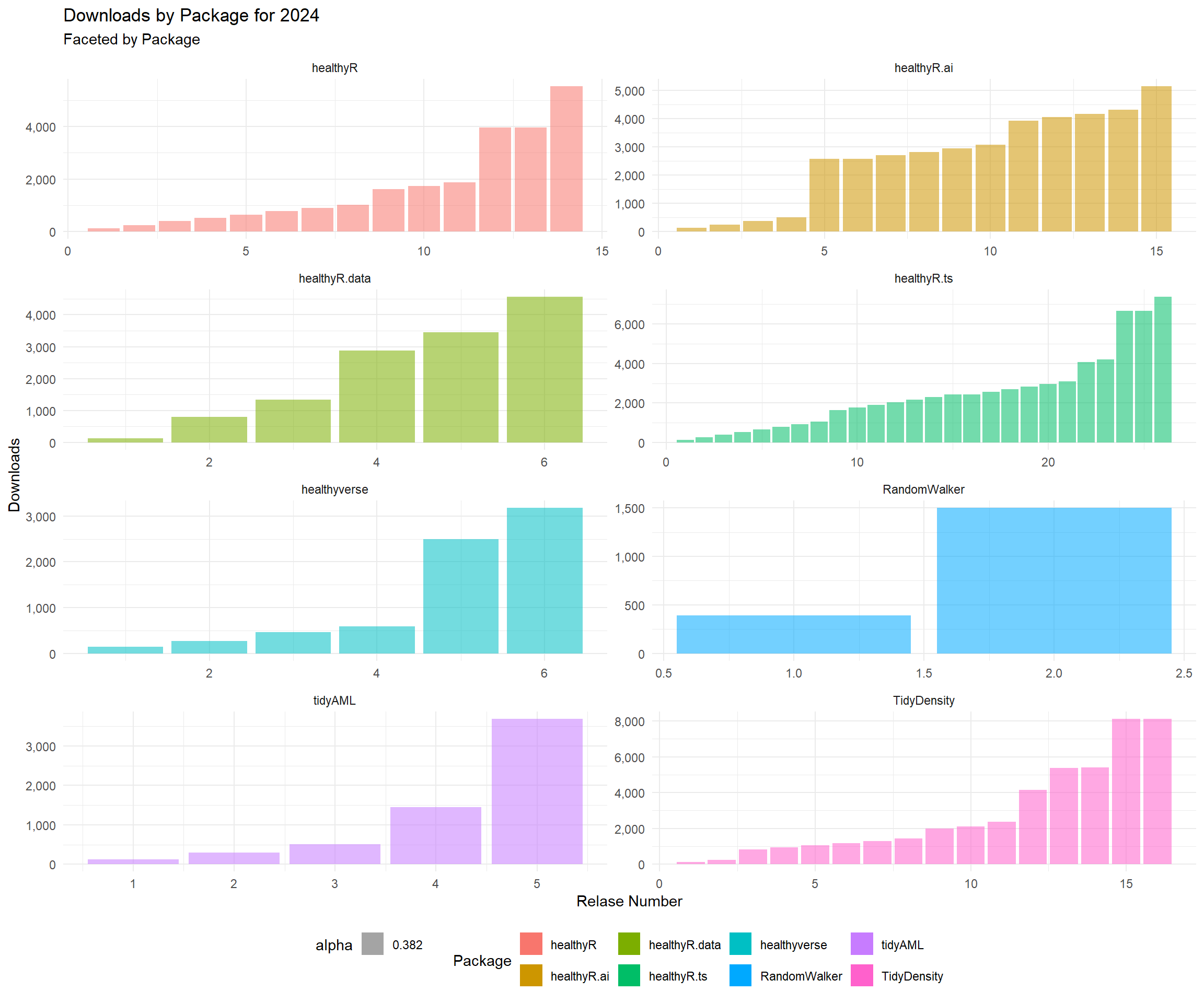``````data_tbl %>%
count(package, version) %>%
group_by(package) %>%
mutate(record = row_number()) %>%
ungroup() %>%
geom_line(aes(color = package, group = package), size = 1) +
scale_y_continuous(labels = scales::label_number(big.mark = ",")) +
theme_minimal() +
theme(legend.position = "bottom") +
labs(
subtitle = "Colored by Package",
x = "Release Number",
color = "Package"
)``````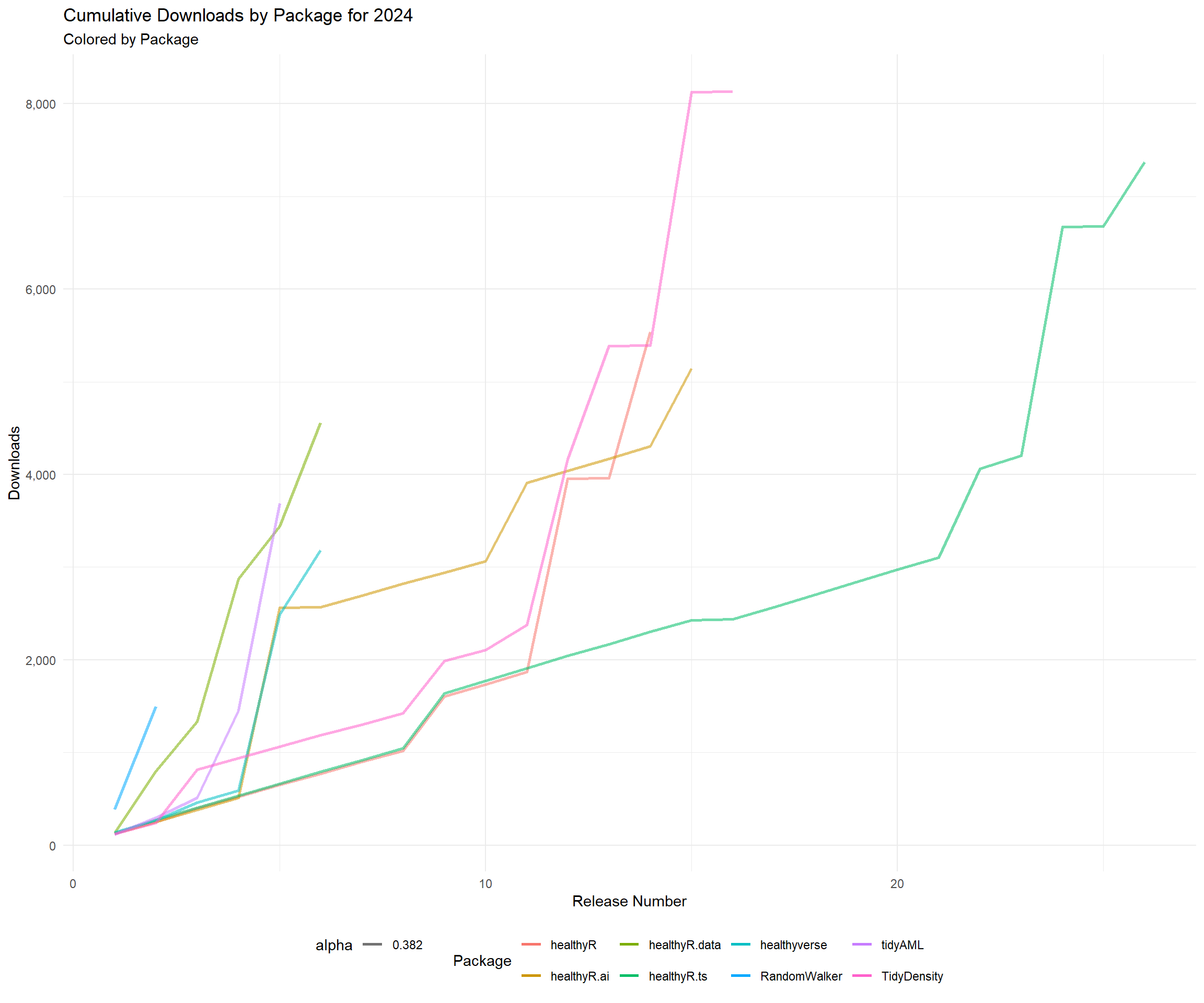# Time Series Graphs

Now lets get some time-series graphs.

``````library(healthyR.ts)

data_tbl %>%
summarise_by_time(.date_var = date, n = n()) %>%
ts_calendar_heatmap_plot(.date_col = date, .value_col = n, .interactive = FALSE)``````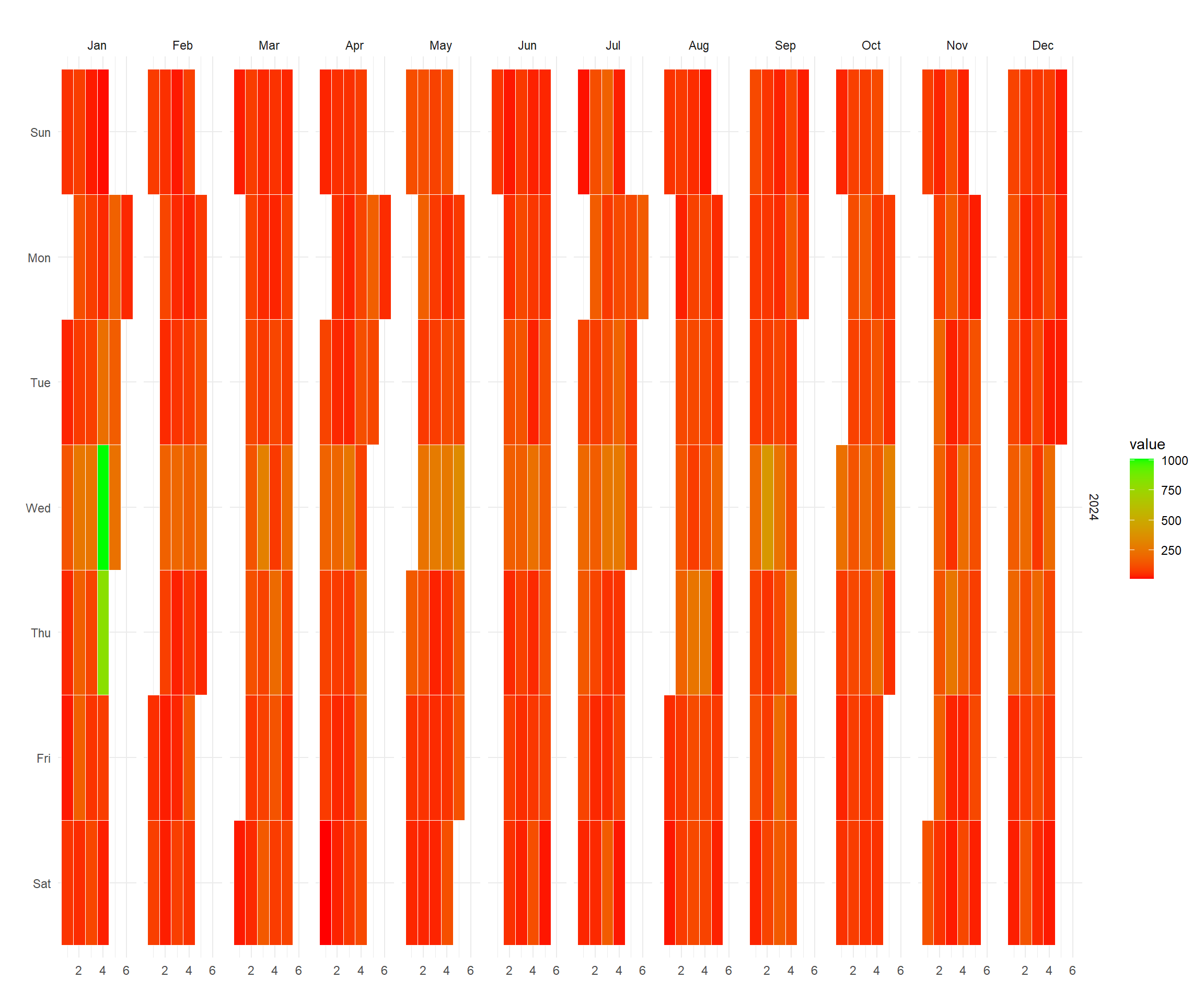``````data_tbl %>%
ggplot(aes(date, log1p(value))) +
geom_point(aes(group = package, color = package), size = 1) +
geom_smooth(method = "loess", color = "black",  se = FALSE) +
geom_vline(
data = pkg_tbl
, aes(xintercept = as.Date(date))
, color = "red"
, lwd = 1
, lty = "solid"
) +
facet_wrap(package ~., ncol = 2, scales = "free_x") +
theme_minimal() +
labs(
subtitle = "Vertical lines represent release dates",
x = "Date",
y = "log1p(Counts)",
color = "Package"
) +
theme(legend.position = "bottom")``````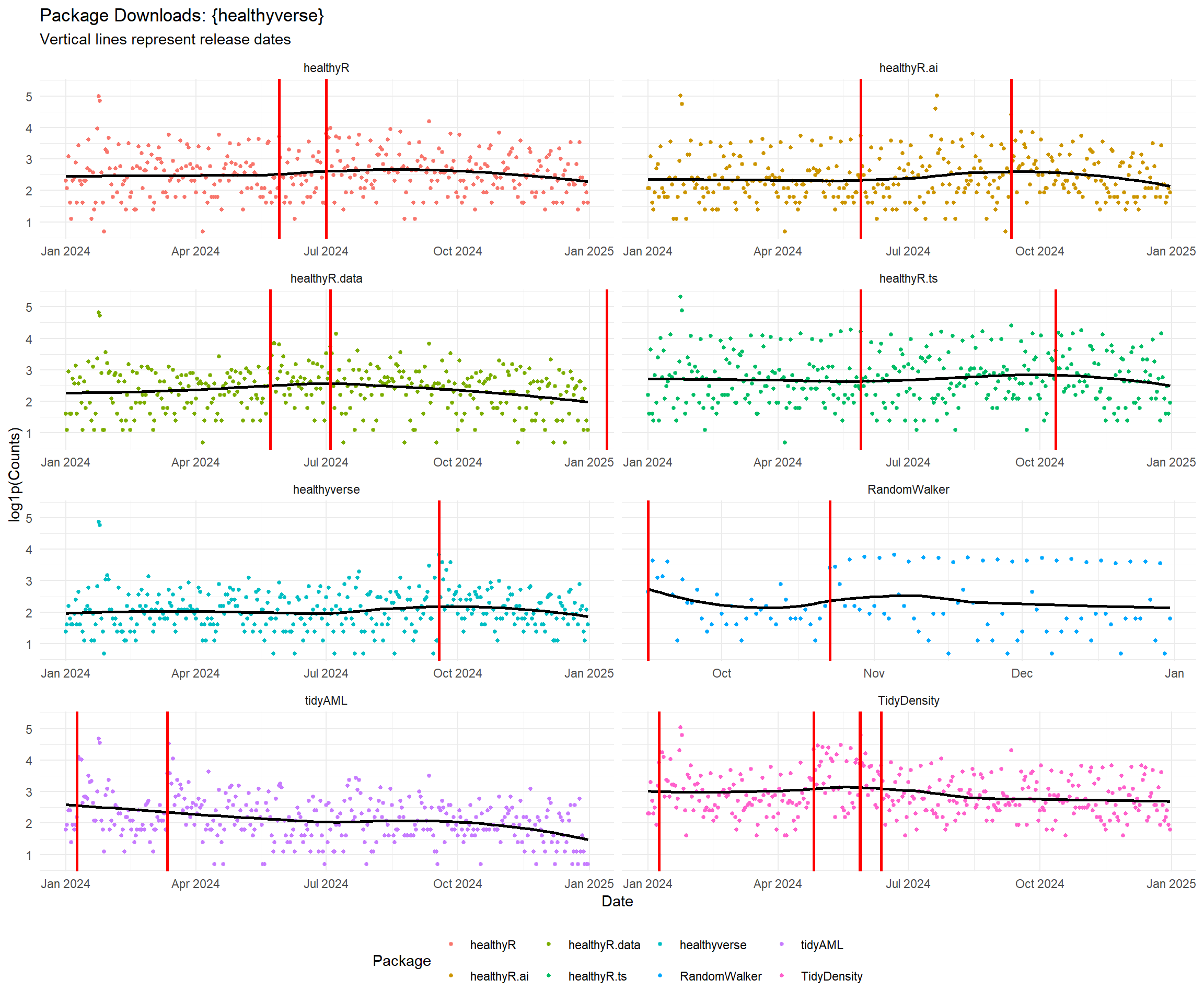``````data_tbl %>%
rename(Actual = value) %>%
tk_augment_differences(.value = Actual, .differences = 1) %>%
tk_augment_differences(.value = Actual, .differences = 2) %>%
rename(velocity = contains("_diff1")) %>%
rename(acceleration = contains("_diff2")) %>%
pivot_longer(-date) %>%
mutate(name = str_to_title(name)) %>%
mutate(name = as_factor(name)) %>%
ggplot(aes(x = date, y = log1p(value), group = name)) +
geom_point(alpha = .2) +
geom_vline(
data = pkg_tbl
, aes(xintercept = as.Date(date), color = package)
, lwd = 1
, lty = "solid"
) +
facet_wrap(name ~ ., ncol = 1, scale = "free") +
theme_minimal() +
labs(
subtitle = "Vertical Lines Indicate a CRAN Release date for a package.",
x = "Date",
y = "",
color = ""
) +
theme(legend.position = "bottom")``````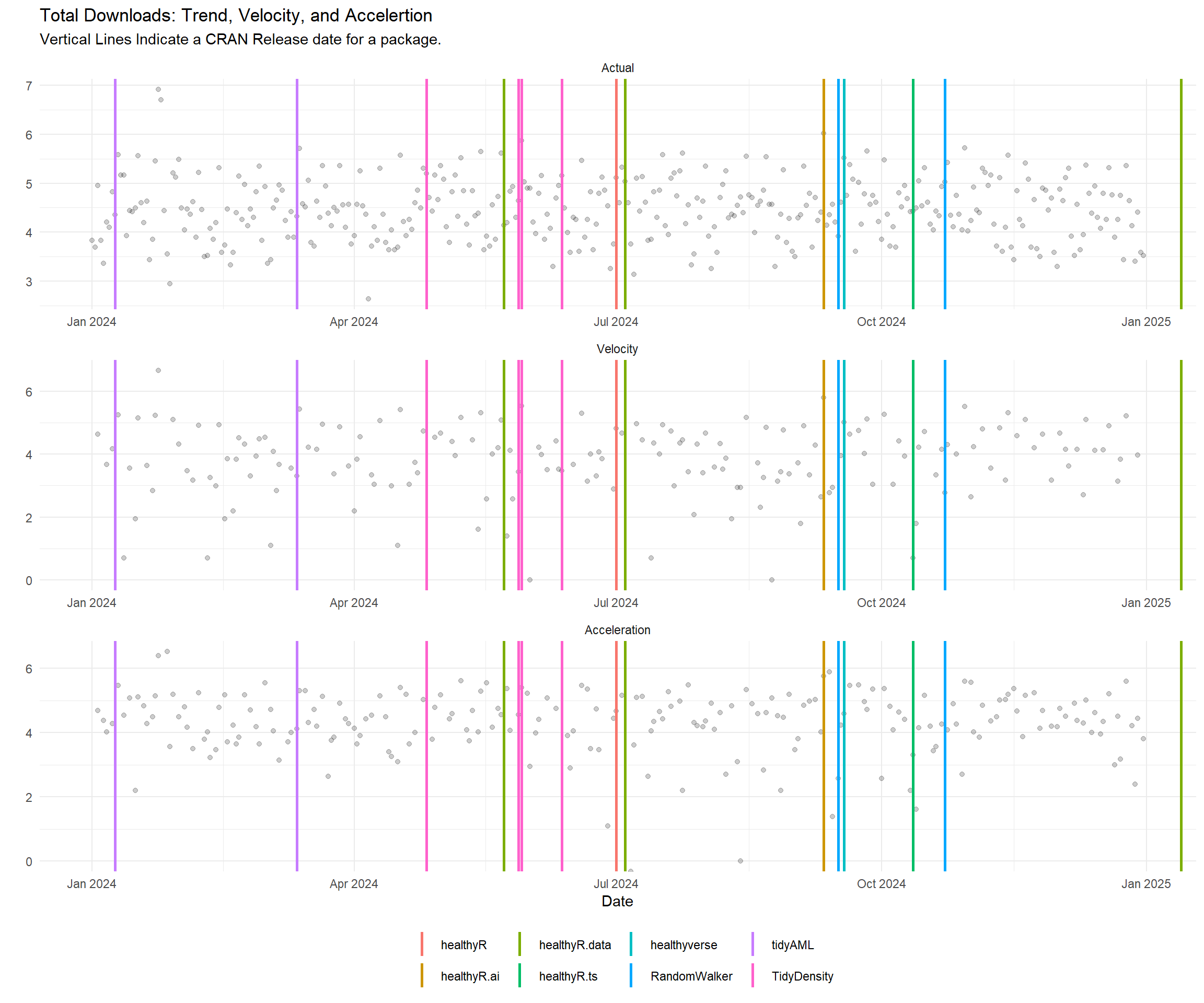``````data_tbl %>%
plot_seasonal_diagnostics(
.date_var = date,
.value = log1p(value),
.interactive = FALSE
) +
theme_minimal() +
labs(
title = "Seasonal Diagnostics",
subtitle = "Values are log1p"
)``````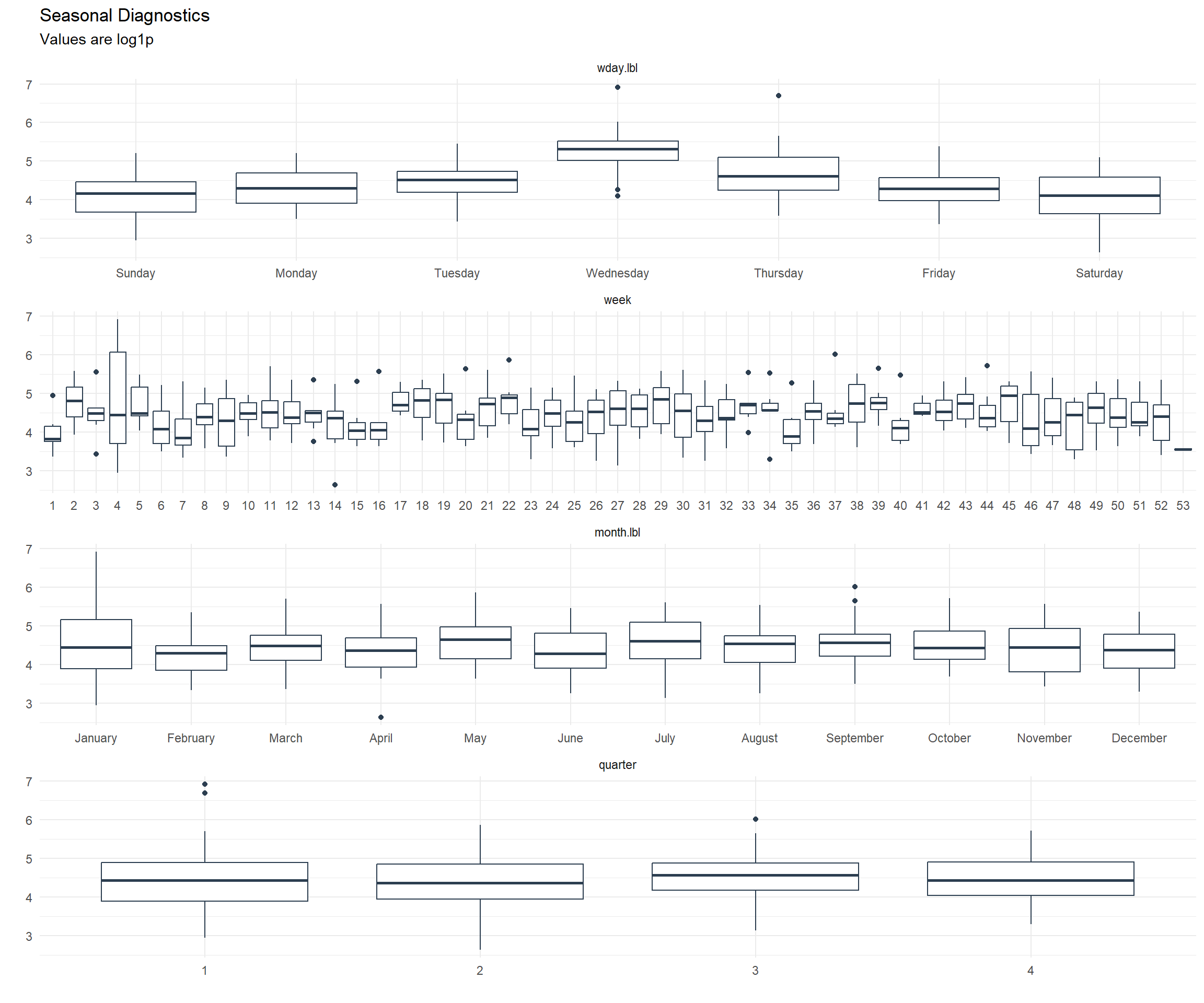``````data_tbl %>%
plot_stl_diagnostics(
.date_var = date,
.value = log1p(value),
.interactive = FALSE
) +
theme_minimal() +
labs(
title = "STL Diagnostics",
subtitle = "Values are log1p"
)``````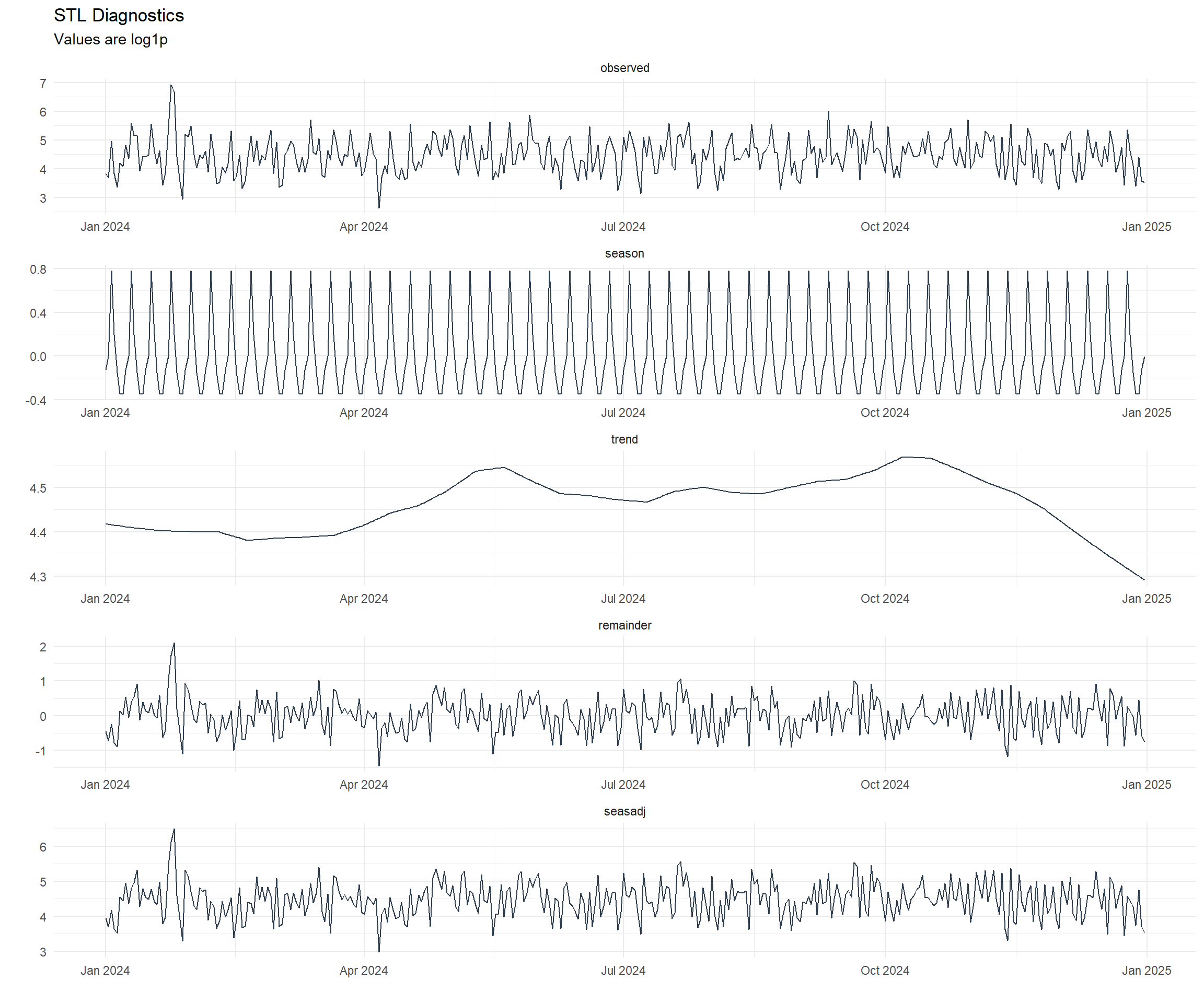# Mapping

So now that we have seen all the downloads in variaous ways, where did they all come from? Lets take a look.

``````library(tmaptools)
library(countrycode)
library(mapview)
library(htmlwidgets)
library(webshot)

mapping_dataset(.data_year = "2022") %>%
knitr::kable()``````
country latitude longitude display_name icon
United States 39.783730 -100.445882 United States https://nominatim.openstreetmap.org/ui/mapicons//poi_boundary_administrative.p.20.png
Hong Kong SAR China 22.350627 114.184916 香港 Hong Kong, 中国 https://nominatim.openstreetmap.org/ui/mapicons//poi_boundary_administrative.p.20.png
``````# l <- map_leaflet(.data = data_tbl)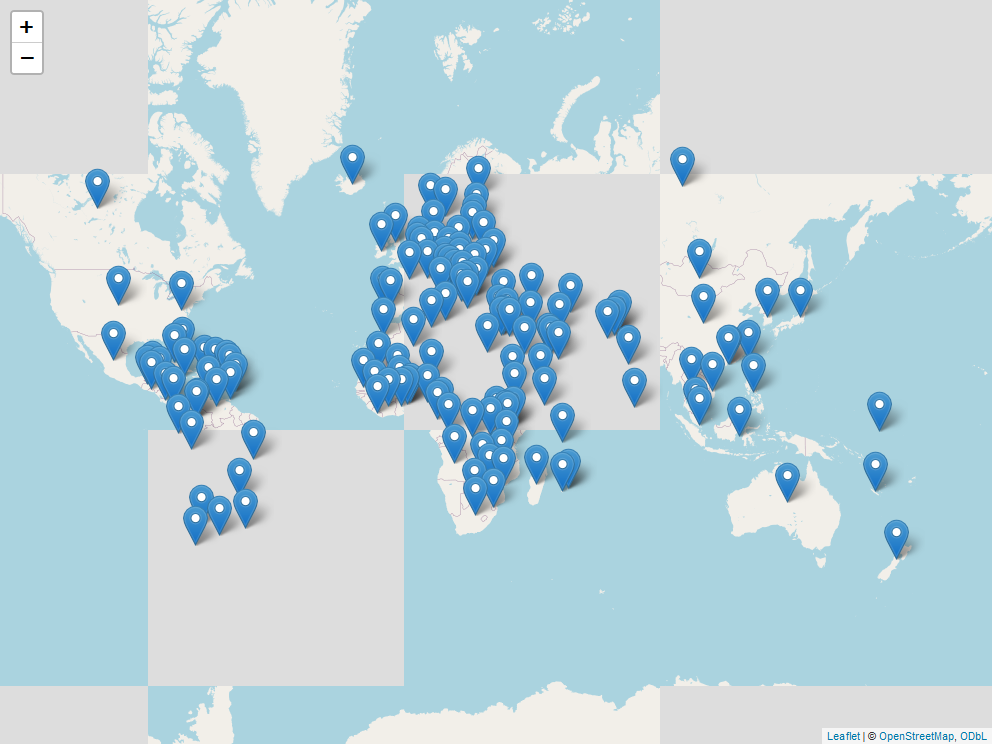There was a total of 121 different countries that downloaded `healthyverse` packages in 2022.